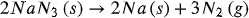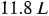1
149
views
45
Problem

# Problem 45

## Chapter 5: GasesTextbook ExpertVerified Tutor
25 Oct 2021

#### Given information

The balanced chemical equation for the given reaction is,The volume of nitrogen gas formed in the reaction is.

#### Step-by-step explanation

Step 1.

Calculate the number of moles of nitrogen by using the ideal gas equation.